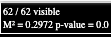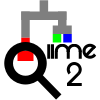# Procrust analysis questions

Hi all,

After searching the forum for similar questions, I still need some guidance regarding the output of procrust analyses in Qiime2.

I was able to run the qiime diversity procrustes-analysis plugin and one of the outputs is called “disparity-results”. It is a qza file, so I´d like to ask if it is possible to visualize its contents?

After that, I used the qiime emperor procrustes-plot plugin. For that, I used the following command:
qiime emperor procrustes-plot
–i-reference-pcoa Pcoa1/unweighted_unifrac_pcoa_results.qza
–i-other-pcoa Pcoa2/unweighted_unifrac_pcoa_results.qza
–i-m2-stats Procrust/pcoa_distance_disparity.qza
–o-visualization Procrust/procrust-plot.qzv

1. For the qiime emperor procrustes-plot command, should I use the original pcoa files or the “normalized pcoa files” generated by the qiime diversity procrustes-analysis? For now, I have used the original pcoa files, as described above.
2. Regarding the M^2 and p-value shown in the emperor procrustes-plot, is this interpretation correct?
M^2 = minimization of residual sum of squares after matching. p = Monte Carlo p-value.

FS

Hi @fstudart!

There are two ways. The first, is using `qiime emperor procrustes-plot`, as you have listed above. The stats will show up in the upper left corner of the plot:The second way is to run `metadata tabulate`:

`````` qiime metadata tabulate \
--m-input-file Procrust/pcoa_distance_disparity.qza \
--o-visualization Procrust/pcoa_distance_disparity.qzv
``````

Either approach will produce the same results.

My understanding is that you should use the same `reference-pcoa` and `other-pcoa` that you used in the `diversity procrustes-analysis` step.

The M^2 value is computed as described here:

https://docs.scipy.org/doc/scipy/reference/generated/scipy.spatial.procrustes.html#scipy.spatial.procrustes

The p value is computed as described here:

The p-value is computed by calculating the M^2 value after switching the row order one input matrix in Monte Carlo simulations, then calculating the number of reordered matrices have a lower M^2 value than the true matrices.

I hope that helps!Hi @thermokarst ,

It definitely helps. Thanks a lot for the feedback.
The metadata tabulate command actually helped me a lot.
Just one additional question: I checked the link you mentioned, and it says: “Procrustes analysis, a similarity test for two data sets.” Thus, if the p-value from the qiime diversity procrustes-analysis is < 0.05, it means that both pcoa files are similar, which reached statistical significance. Conversely if the p-value is > 0.05, the pcoa files are actually dissimilar. Would this be correct?

Thanks,
FS

1 Like

I think so, although I chatted with @jwdebelius out-of-band and she suggested also running a mantel test, too - the more perspectives you have, the better you can support your analysis. You can run mantel on the distance matrices used to generate your PCoA outputs, using `diversity mantel`. I hope that helps!

1 Like

This topic was automatically closed 31 days after the last reply. New replies are no longer allowed.